# Leveraging baseline covariates for improved efficiency in randomized controlled trials

In a previous post I talked about the issue of covariate adjustment in randomized controlled trials, and the potential for improving the precision of treatment effect estimates. In this post I’ll look at one of the (fairly) recently developed approaches for improving estimates of marginal treatment effects, based on semiparametric theory.

Marginal versus conditional treatment effects
In the previous post on the topic, we noted the fact that apart from special cases (e.g. continuous outcomes modelled by linear regression), the true adjusted and unadjusted treatment effect parameters (i.e. the true population values) differ. This is despite the fact that in a randomized controlled trial confounding is not an issue – randomization guarantees us that, in expectation, the two treatment groups are balanced with respect to all factors, both measured and unmeasured.

An important example where the unadjusted and adjusted treatment effects differ is when logistic regression is used to model a binary outcome. That is, the marginal or unadjusted odds ratio for the effect of treatment differs from the treatment effect conditional on one or more baseline covariates. This means that if one adjusts for baseline measurements, the true treatment effect estimate is actually different from the marginal unadjusted treatment effect. It turns out that conditional (adjusted) odds ratios for treatment are larger in absolute magnitude than the marginal (unadjusted) effect.

Both the unadjusted odds ratio and adjusted (for a given set of baseline covariates) are legitimate parameters which may be interest. However, as noted in my previous post, two issues which arise with the adjusted effect are that estimates are only unbiased (asymptotically) if we include the baseline covariates in the logistic regression model correctly (i.e. include in the correct functional forms, and include interactions where they exist), and that the true adjusted treatment effect will differ between trials if they adjust for different sets of baseline covariates.

An advantage of the unadjusted (marginal) odds ratio is that its meaning is unambiguous, and because we are not adjusting for any baseline covariates, there are no concerns about mis-specification of the logistic regression model. However, as noted in the previous post, the drawback of such an approach is that baseline information which is (usually) available is not exploited. Recently new approaches have been developed which enable such baseline information to be use to obtain estimates of the marginal odds ratio which are more precise than the one from the unadjusted logistic regression. Furthermore, unlike the adjusted logistic regression approach, these new approaches provide unbiased estimates regardless of whether the way in which the baseline covariates have been adjusted for is correct.

Estimating the marginal treatment effect with improved precision
In this post I’ll look at one of these new approaches, based on semiparametric theory. The paper by Zhang et al developing the approach can be found here, and a nice slide presentation detailing the methodology by Marie Davidian can be found here.

The basic idea is that we can modify the estimating equations which are solved by the marginal (unadjusted) treatment effect estimator by adding an augmentation function, which makes use of the baseline covariates. The construction of the augmentation function depends on specifying a parametric working model for predicting the outcome using the baseline covariates. Importantly however, estimates remain (asymptotically) unbiased irrespective of whether this so called working model is correctly specified.

In this post I’ll consider the case of a binary outcome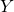in a two-arm trial, where interest lies in estimating the marginal odds ratio: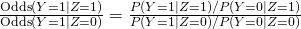Here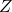is a binary variable indicating which treatment group a subject is randomized to. We will let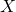denote a vector of baseline covariates.

To obtain an estimate of the marginal odds ratio with improved efficiency, we have to specify a model for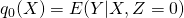and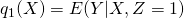, that is models for the expectation of the outcome given the baseline covariates, in each of the two treatment arms. Since we are assumingis binary here, we’ll use logistic regression models for these two models. Note that we could potentially include different covariates in the two groups, or different functional forms or interactions.

We can then fit these, separately in the two groups. Next, we must calculate the predicted values offor every subject, first assuming they are in the control group (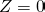), and then in the intervention group (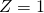). That is,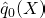and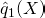. These values are then used to construct the augmentation function, and consequently the augmented estimating equations, which are solved to obtain the treatment effect estimate (see paper).

Implementation in R
Fortunately an R package, speff2trial, has been developed by Michal Juraska implementing the approach for binary and censored time-to-event outcomes. To illustrate its use, we’ll simulation some data for a simple trial with a single baseline covariate:

set.seed(65456461)
n <- 1000
z <- 1*(runif(n) < 0.5)
x <- rnorm(n)
xb <- -2+x+z
prob <- exp(xb)/(1+exp(xb))
y <- 1*(runif(n) < prob)
data <- data.frame(y,x,z)


Here the true conditional treatment effect log odds ratio is 1. The true marginal log odds ratio will be some value smaller than 1, since conditional effects are always larger than marginal (in the absence of confounding).

First, let's fit the simple unadjusted model to estimate the marginal treatment effect but without using the baseline covariate:

> unadjusted <- glm(y~z, data, family=binomial)

Call:
glm(formula = y ~ z, family = binomial)

Deviance Residuals:
Min       1Q   Median       3Q      Max
-0.8753  -0.8753  -0.6324   1.5132   1.8482

Coefficients:
Estimate Std. Error z value Pr(>|z|)
(Intercept)  -1.5080     0.1199 -12.580  < 2e-16 ***
z             0.7462     0.1518   4.915 8.86e-07 ***
---
Signif. codes:  0 ‘***’ 0.001 ‘**’ 0.01 ‘*’ 0.05 ‘.’ 0.1 ‘ ’ 1

(Dispersion parameter for binomial family taken to be 1)

Null deviance: 1133.4  on 999  degrees of freedom
Residual deviance: 1108.3  on 998  degrees of freedom
AIC: 1112.3

Number of Fisher Scoring iterations: 4


The estimated log odds ratio for the intervention vs control contrast is 0.746, standard error 0.152, and z-statistic 4.915. Now let's perform the standard adjusted analysis, includinginto the linear predictor of the logistic regression:

> adjusted <- glm(y~z+x, data, family=binomial)

Call:
glm(formula = y ~ z + x, family = binomial)

Deviance Residuals:
Min       1Q   Median       3Q      Max
-2.3303  -0.7392  -0.4792   0.6146   2.5612

Coefficients:
Estimate Std. Error z value Pr(>|z|)
(Intercept) -1.88117    0.14173 -13.273  < 2e-16 ***
z            0.94074    0.16850   5.583 2.36e-08 ***
x            1.07543    0.09532  11.282  < 2e-16 ***
---
Signif. codes:  0 ‘***’ 0.001 ‘**’ 0.01 ‘*’ 0.05 ‘.’ 0.1 ‘ ’ 1

(Dispersion parameter for binomial family taken to be 1)

Null deviance: 1133.37  on 999  degrees of freedom
Residual deviance:  941.29  on 997  degrees of freedom
AIC: 947.29

Number of Fisher Scoring iterations: 5


The adjusted conditional treatment effect estimate is 0.941, standard error 0.169, and z-statistic 5.583. As we should expect (on average), the conditional treatment effect is larger in magnitude than the marginal effect. Despite adjusting for baseline, the standard error is increased, but this is because we now estimating a larger parameter value. Note however that the z-statistic is larger - the test has increased power compared to the unadjusted analysis.

Now we'll estimate the marginal treatment effect, but leveraging the baseline covariateto obtain a more precise estimate. To do this we first (install) and load the speff2trial package:

library(speff2trial)


Next, we must fit the two working modelsand. To do this we fit separate logistic regression models in the two treatment groups:

q0mod <- glm(y[z==0]~x[z==0], data, family=binomial)
q1mod <- glm(y[z==1]~x[z==1], data, family=binomial)


Now we need to calculateandfor every subject, that is the predicted probability of a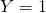outcome, given, under both control and intervention conditions. To do this we can use the following code:

expit <- function(linpred){
exp(linpred)/(1+exp(linpred))
}
q0hat <- expit(cbind(rep(1,n),x) %*% q0mod$coef) q1hat <- expit(cbind(rep(1,n),x) %*% q1mod$coef)


The first part just defines a function which is the inverse of the logit. The predicted probabilities are then found by calculating the linear predictor values from the two models, and applying the expit function.

Lastly, we call the speff function:

semiPara <- speff(y ~ 1, endpoint="dichotomous", data, trt.id="z",
endCtrlPre=q0hat, endTreatPre=q1hat)


We first specify the outcome variable y, and don't put any variables on the right hand side of the equation (although see later on variable selection). We then tell the function that the outcome is binary/dichotomous, pass the data frame, identify the treatment variable, and then pass the predicted values we have just generated. To obtain the treatment effect estimate we just summarise the fitted object:

> summary(semiPara)

Treatment effect
Log OR       SE       LB      UB           p
Naive  0.74625  0.15182  0.44869  1.0438  8.8591e-07
Speff  0.78412  0.13967  0.51036  1.0579  1.9771e-08


We first are given the so called 'naive' marginal treatment effect estimate which does not utilize the baseline covariate(s) (I'm not sure I would call it a naive estimate, it's just not efficient). Next we have the estimated marginal log odds ratio which utilizes the baseline covariate(s). As we would hope from the theory, the standard error is smaller, the p-value more significant, and the confidence interval is narrower - we have gained precision / statistical efficiency by using the baseline covariate.

Model selection
In the preceding analysis we have specified the form of the functionsand. Zhang et al state that we can use standard model selection techniques (e.g. stepwise selection) to choose these models. Provided this is performed separately in the two arms, asymptotically this covariate selection step does not affect the validity of inferences.

The speff function's default approach is actually to perform variable selection using the baseline covariates which are passed to it. In this case, we do not pass the predicted values, but let the function select the baseline covariates which best predict the outcome in each treatment group. A number of variable selection approaches are available in the function, and also different choices of what measure of model performance is used to pick the best model. In the above example we had only a single covariate, so there isn't really any variable selection to do.

Ordinarily if baseline covariates are adjusted for, this must be prespecified in the analysis plan in advance. This is to avoid baseline covariate adjustment to be chosen on the basis of giving the 'best' treatment effect, potentially introducing bias and increase type 1 error rate. However, since the modelsandare developed separately in each arm, and without reference to what the estimated treatment effect is, this shouldn't be an issue. Indeed in an earlier paper Tsiatis argued that two sets of analysts might be used, one to develop themodel using the control data and another to developin the intervention arm, to allay fears about lack of pre-specification.

However, I suspect that some investigators may still prefer to pre-specify which baseline covariates will be adjusted for and how they will be included in the working models. There is of course no problem with this - all that is lost is that the resulting treatment effect estimate is potentially not as efficiency as it might be. Again it is important to emphasize that mis-specification of these working models does not introduce bias in the treatment effect estimator, but instead some efficiency is lost.

One last point. This approach relies on asymptotic arguments for asymptotic unbiasedness and also for the standard error estimation using the sandwich approach. Thus I would be cautious about using it in 'small' studies. How small is small? In Zhang et al's paper simulations were performed using n=600, and the estimates were unbiased and confidence intervals had their nominal 95% coverage. Thus the approach seems perfectly viable with such a study size (and therefore of course larger study sizes too).

### 2 thoughts on “Leveraging baseline covariates for improved efficiency in randomized controlled trials”

1.Hi, I have a question that relates to observational studies. In a comparative study, baseline covariates may be adjusted for in order to accurately measure treatment effect for comparison with another intervention. However, in a single-arm study (e.g. looking at the effect of an intervention from baseline) is it still important to account for baseline variables?

•